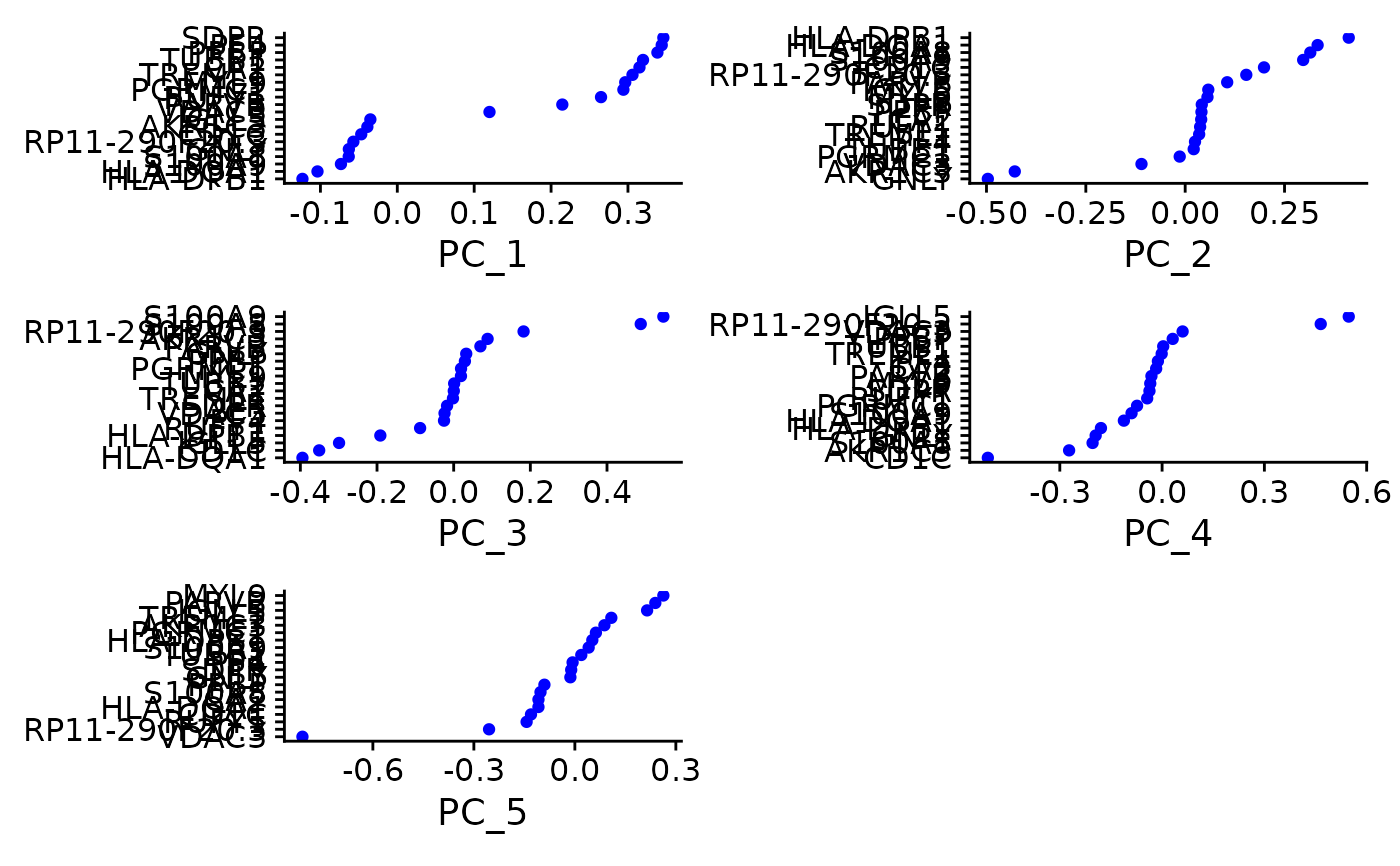Visualize top genes associated with reduction components

VizDimLoadings(
object,
dims = 1:5,
nfeatures = 30,
col = "blue",
reduction = "pca",
projected = FALSE,
balanced = FALSE,
ncol = NULL,
combine = TRUE
)

## Arguments

object

Seurat object

dims

Number of dimensions to display

nfeatures

Number of genes to display

col

Color of points to use

reduction

Reduction technique to visualize results for

projected

Use reduction values for full dataset (i.e. projected dimensional reduction values)

balanced

Return an equal number of genes with + and - scores. If FALSE (default), returns the top genes ranked by the scores absolute values

ncol

Number of columns to display

combine

Combine plots into a single patchwork ggplot object. If FALSE, return a list of ggplot objects

## Value

A patchwork ggplot object if combine = TRUE; otherwise, a list of ggplot objects

## Examples

data("pbmc_small")